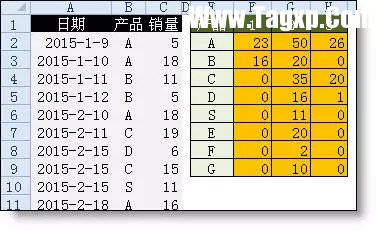#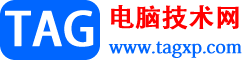## 常用的excel函数公式大全

一、数字处理

1、取绝对值

=ABS(数字)

2、取整

=INT(数字)

3、四舍五入

=ROUND(数字,小数位数)

1、把公式产生的错误值显示为空

=IFERROR(A2/B2,"")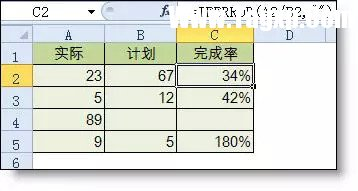2、IF多条件判断返回值

=IF(AND(A2<500,B2="未到期"),"补款","")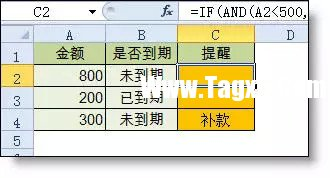1、统计两个表格重复的内容

=COUNTIF(Sheet15!A:A,A2)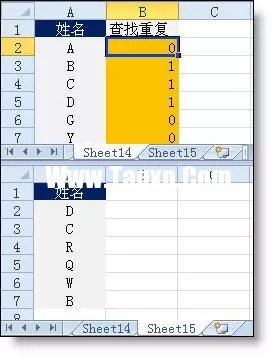2、统计不重复的总人数

=SUMPRODUCT(1/COUNTIF(A2:A8,A2:A8))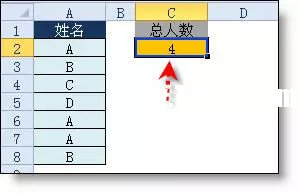1、隔列求和

=SUMIF(\$A\$2:\$G\$2,H\$2,A3:G3)

=SUMPRODUCT((MOD(COLUMN(B3:G3),2)=0)*B3:G3)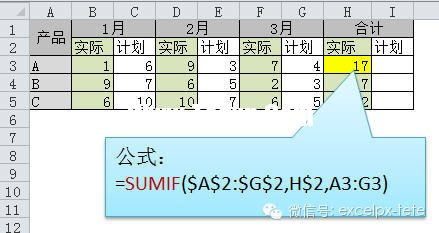2、单条件求和

=SUMIF(A:A,E2,C:C)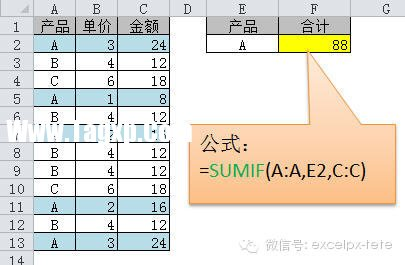3、单条件模糊求和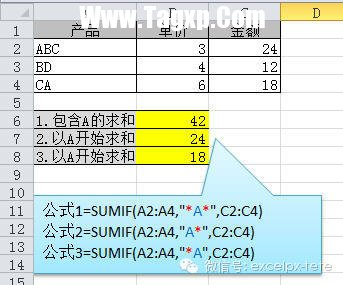4、多条件模糊求和

=SUMIFS(C2:C7,A2:A7,A11&"*",B2:B7,B11)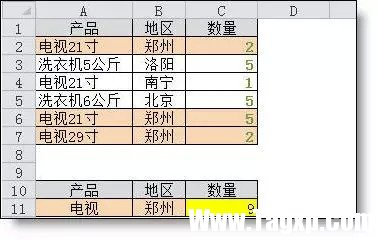5、多表相同位置求和

=SUM(Sheet1:Sheet19!B2)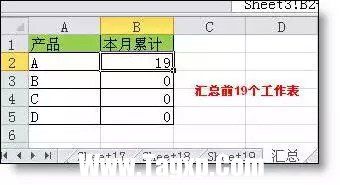6、按日期和产品求和

=SUMPRODUCT((MONTH(\$A\$2:\$A\$25)=F\$1)*(\$B\$2:\$B\$25=\$E2)*\$C\$2:\$C\$25)